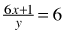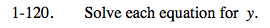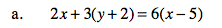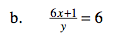### Home > PC > Chapter 1 > Lesson 1.3.2 > Problem1-120

1-120.
1. Solve each equation for y. Homework Help ✎

1. 2x + 3(y + 2) = 6(x − 5)

2.Distribute and simplify.y cannot be equal to 0. Why?

$y = x + \frac{1}{6}$

Why is this true?

$x\neq -\frac{1}{6}$

Then y = 0 and you would have division by 0 or undefined.